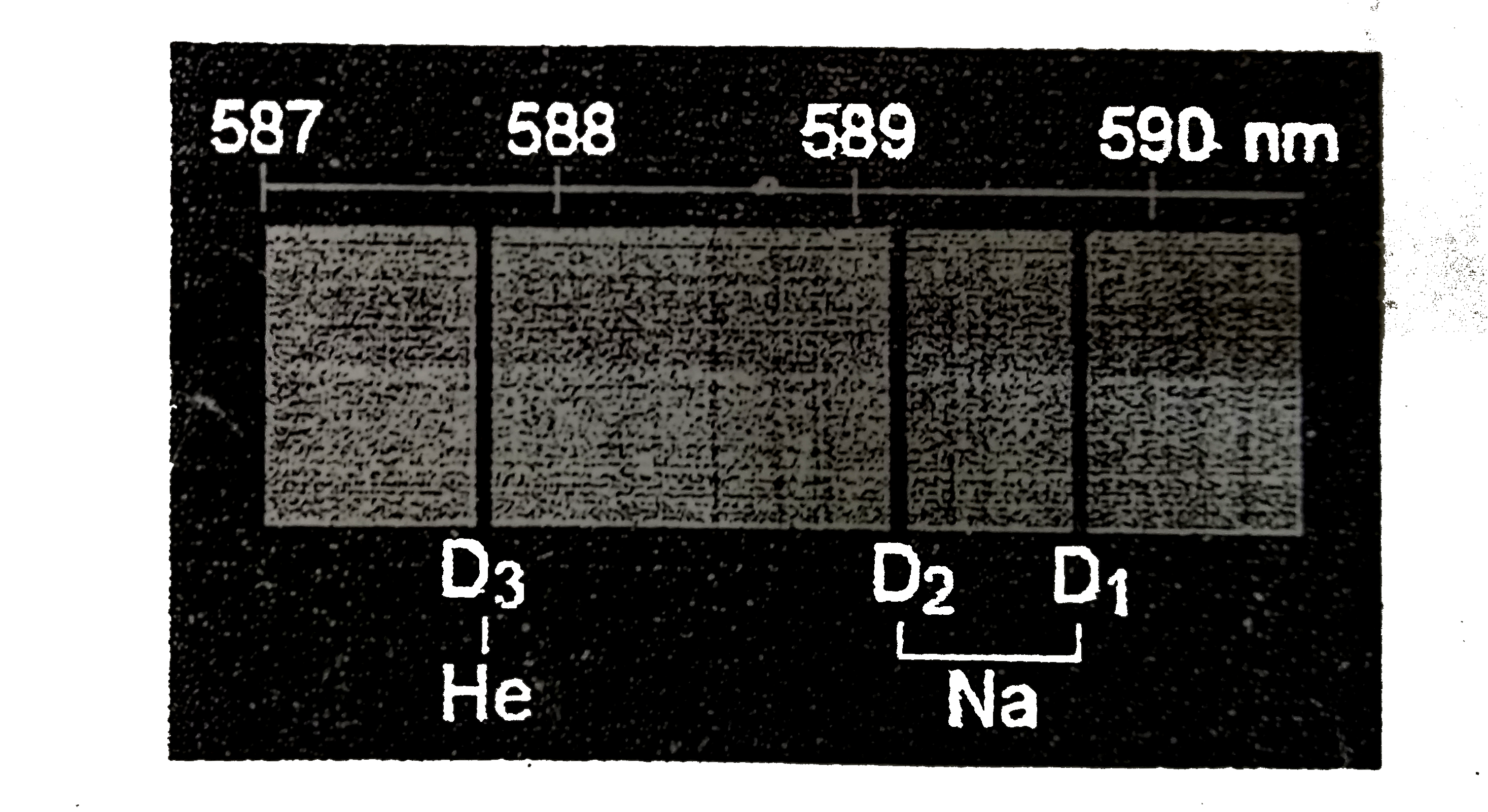# In 1894, Lord Rayleigh reported that the mass of chemically prepared nitrogen was different from that of nitrogen extracted from the atmosphere, as

10 views
In 1894, Lord Rayleigh reported that the mass of chemically prepared nitrogen was different from that of nitrogen extracted from the atmosphere, as shown in Tables 1 and 2. Latter, this difference was attributed to the presence of argon in atmospheric nitrogen. The masses of gases were mearsured by using a glass vessel with a known volume under atmospheric pressure (1.013xx10^(5)Pa).
|{:("From nitric oxide",,2.3001 g),("From nitrous oxide",,2.2990 g),("From amonium nitrite purified at a red heat",,2.2987 g),("From urea",,2.2985 g),("From ammonium nitrite purified in the cold",,2.2987 g),("Mean",,2.2990 g):}|
|{:(O_(2) "was removed by hot copper" (1892),,2.3103 g),(O_(2) "was removed by hot iron" (1893),,2.3100 g),(O_(2) "was removed by ferrous hydrate (1894),2.3102 g,),(Mean,,2.3102 g):}|
Ramsay and cleve discovered helium in cleveite (a mineral consiting of uranium oxide and oxides of lead, thorium, and rare earths, an impure variety of uraninite) independently and virtually simultaneously in 1895. The gas extracted from the rock showed a unique spectroscopic line at around 588 nm (indicated by D3 in Figure 1), which was fist observed in the spectrum of solar prominence during a total eclipse in 1868, near the well-known D_(1) and D_(2) lines of sodium.ul("Which") equation explains the occurrence of argon in rocks among [A] to [D] below? Mark one.
One of the strongest evidences for the monoatomicity of argon and helium is the ratio of the heat capacity under constant pressure to that at constant volume, gamma=C_(p)//C_(v), which is exactly 5//3 (1.67 +- 0.01) for a monoatomic gas. The ratio was derived from the measurement of speed of sound v_(s) by using the following equation, where f and the frequency and wavelength of the sound, and R, T, and M denote the molar gas constant, absolute temperature, and molar mass, respectively.
v_(s)=flambda=sqrt((gammaRT)/(M))
For an unknown gas sample, the wavelength of the sound was measured to be =0.116m at a frequency of f=3520 Hz (Hz =s^(-1)) and temperature of 15.0^(@)C and under atmospheric pressure (1.013. 10^(5) Pa). The density of the gas for these conditions was measured to be 0.850 +- 0.005 kg m^(-3).
A. ArF_(2)" " Ar+F_(2)
B. ArXe rarr Ar+Xe
C. ^(40)K rarr ^(40)Ar+ epsilon//beta^(+) (electron capture//position emission)
D. ^(126)I rarr ^(126)Ar+beta^(-)

by (73.0k points)
Correct Answer - [C] is a well known radioactive decay reaction occurring with a half life of the order of the earth age. No stable compound of Ar, such as ArF_(2) or ArXe, can be expected
Products of [D] should be ^(126)Xe+beta^(-). The correct answer is [C]